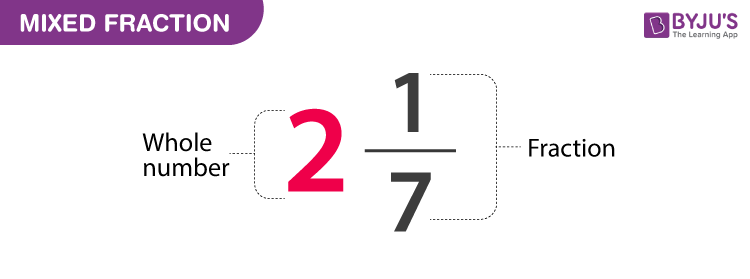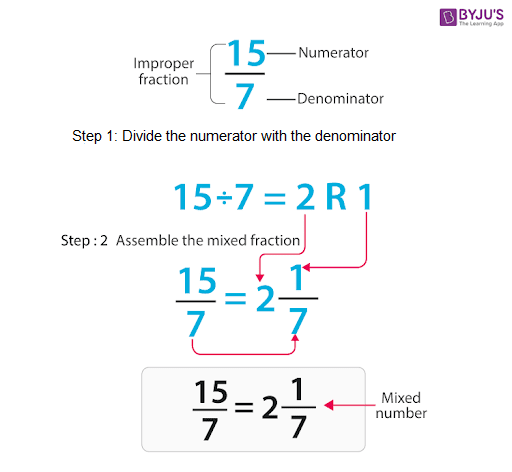# Mixed Fractions

Mixed Fractions are one of the three types of fractions. It is also called mixed numbers. For example, 21/7 is a mixed fraction. Learn here all types of fractions in detail.

You can understand these fractions in details in this article, such as its definition, changing of the improper fraction to a mixed fraction and so on. Also, you will learn here to perform operations like multiplying, dividing, adding and subtracting fractions. Read the complete article to become well versed with all the related concepts of these types of fractions.

## Definition

It is a form of a fraction which is defined as the ones having a fraction and a whole number.

Example2(1/7), where 2 is a whole number and 1/7 is a fraction.## How to convert Improper fraction to a mixed fraction?

• Step 1: Divide the Fraction’s numerator with the denominator, i.e. 15/7.
• Step 2: The integer part of the answer will be the integer part for a mixed fraction, i.e. 2 is an integer.
• Step 3: The Denominator will be the same as original, i.e 7.
• Step 4: So, the improper fraction 15/7 is changed to a Mixed fraction as 2 (1/7)Some more examples of mixed fractions are 3(¼), 1 (2/9), 7(¾).

## Mixed fraction to Improper Fraction

• Step 1: Multiply the denominator with the whole number, i.e. Multiply 7 with 2 in the given example, 2(1/7).

7 × 2  =14

• Step 2: Add the numerator of the Fraction to the result in step 1. i.e., Add 1+ 14

=15.

• Step 3: Keep the Denominator same i.e. 7.
• Step 4: The Improper fraction obtained is: 15/7.

When it comes to adding Mixed or Improper fractions, we can have either the same denominators for both the fractions to be added or the denominators can differ too.

Here’s a step-wise method to add the improper fraction with same or different denominators.

Note: Before applying any operations such as addition, subtraction, multiplication, etc., change the given mixed fractions to improper fractions as shown above.

 Adding with the same Denominators. Example: 6/4 + 5/4 Adding with the Different Denominators. Example: 8/6 +12 /8 Step 1: Keep the denominator ‘4’ same. Step 1: Find the LCM between the denominators, i.e. the LCM of 6 and 8 is 24 Step 2: Add the numerators ‘6’ +’5’ =11. Step 2: Multiply both Denominators and Numerators of both fractions with a number such that they have the LCM as their new Denominator. Multiply the numerator and Denominator of  8/6 with 4 and 12/8 with 3. Step 3: If the answer is in improper form, Convert it into a mixed fraction, i.e. 11/4  = 2 (¾) Step 3: Add the Numerator and keep the Denominators same. 32 / 24 + 36 / 24   = 68/24 = 17/6 So, We have 2 (¾) wholes. Step 4: If the answer is in Improper form, convert it into Mixed Fraction: 2 (⅚)

## Subtracting Mixed Fractions

Here’s a step-wise explanation of how to Subtract the improper fraction with Same or Different Denominators.

 Subtracting with the same Denominators. Example: 6/4 – 5/4 Subtracting with the different Denominator 12/8 – 8/6 Step 1: Keep the denominator ‘4’ the same. Step 1: Find the LCM between the denominators, i.e. the LCM of 8 and 6 is 24 Step 2: Subtract the numerators ‘6’ -’5’ =1. Step 2: Multiply both Denominators and Numerators of both fractions with a number such that they have the LCM as their new Denominator. Multiply the numerator and Denominator of  8/6 with 4 and 12/8 with 3. Step 3: If the answer is in improper form, Convert it into a mixed fraction. i.e. 1/4 Step 3: Subtract the Numerator and keep the Denominators the same. 36 / 24 – 32/24 = 4/24 So, We have 1/4 wholes. Step 4: If the answer is in Improper form, convert it into Mixed Fraction. 4/24 = 1/6

## Multiplying Mixed Fractions

Example: 2(⅚)  × 3 (½)

Solution:

Step 1: Convert the mixed into an improper fraction. 17/6  × 7/2

Step 2: Multiply the numerators of both the fractions together and denominators of both the fractions together. {17 ×  7} {6 × 2}

Step 3: You can convert the fraction into the simplest form or Mixed one = 119 / 12 or 9 (11/12)

### Definition of Fraction

In simple words, the ratio of the two numbers is called a fraction.

For Example, 15/7  is a fraction, where 15 is a numerator and 7 is a denominator. 7 is the number of parts into which the whole number divides.

A fraction can represent part of a whole.

### Kinds of Fractions

There are three types of fractions. Below given table defines all three of them.

 Types of Fractions Explanation Proper Fraction When the numerator is less than Denominator Improper Fraction When the numerator is greater than the Denominator Mixed Fraction It is an improper function, which is written as a combination of a whole number and a fraction.

## Mixed Equivalent Fractions

How can we find mixed equivalent fractions? Let us find the answer to this question here.

Two fractions are said to be equivalent if their values are equal after simplification. Suppose ½ and 2/4 are two equivalent fractions since 2/4 = ½.

Now when two mixed fractions are equal to each other then they are equivalent in nature. Hence, if we are converting any two equivalent fractions into mixed fractions, then the quotient left, when we divide numerator by denominator, should be the same.

For example, 5/2 and 10/4 are two equivalent fractions.

5/2: when we divide 5 by 2, we get a quotient equal to 2 and the remainder equal to 1. So 5/2 could be written in the form of a mixed fraction as 21/2.

Similarly, in the fraction 10/4, when we divide 10 by 4, we get a quotient equal to 2 and the remainder equal to 2. Therefore, 10/4 = 22/4.

Hence, for both mixed fractions 21/2 and 22/4, the quotient value is equal to 2.

## Video Lesson on Fractions## Frequently Asked Questions – FAQs

Q1

### What is a mixed fraction?

A fraction represented with its quotient and remainder is a mixed fraction. For example, 2 1/3 is a mixed fraction, where 2 is the quotient, 1 is the remainder. So, a mixed fraction is a combination of a whole number and a proper fraction.
Q2

### How to read a fraction?

A fraction denotes a portion of a whole. Therefore, if we have to read a fraction say ¾, then it read as three-fourth of a whole. In the same way, we read the other fractions such as:
½ – half of a whole
¼ – one-fourth of a whole
⅔ – two-thirds of a whole
⅓ – one-third of a whole
Q3

### How to convert an improper fraction into a mixed fraction?

Divide the numerator by denominator.
Take the quotient as a whole number and the remainder as the numerator of the proper fraction, keeping the denominator same.
For example, in 17/3, divide 17 by 3 to get 5 as the quotient and 2 as the remainder. Thus,
17/3 = 52/3
Q4

### How to convert a mixed fraction into an improper fraction?

To convert a mixed fraction into an improper fraction, first, we multiply the denominator of the proper fraction by the whole number attached to it and then we add the numerator.
For example, 31/2 is a mixed fraction.
Multiply 2 and 3, 2×3 = 6
Add 6 and 1(numerator) = 6+1 = 7
Hence, 31/2 = 7/2
Q5

### How to add mixed fractions?

To add two or more mixed fractions, we need to convert them into improper fractions.
Then we need to check if the denominators of the given fractions are equal or not.
If they are equal then we can add them directly, but if they are unequal, we need to find the LCM of denominators and make them equal. Later we can add the numerators, keeping the denominator same.
Q6

### How to subtract mixed fractions?

Subtraction of mixed fractions is the same as the addition method. We need to convert mixed numbers into improper fractions and then subtract them.
Quiz on Mixed Fractions